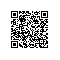# php获分别取数组键名和键值

1. <?php
2. $arr=array 3. "one" => "php" 4. "two" => "java" 5. "three" => array("a" => "apple","b" => "bananner" 6. ); 7. echo '<pre />' 8. print_r(array_keys($arr));//输出键名
9.
10. echo '<pre />'
11. print_r(array_values($arr));//输出键值 12. ?>array_keys() 函数返回包含数组中所有键名的一个新数组。 使用 value 参数： 1. <?php 2.$a=array("a"=>"Horse","b"=>"Cat","c"=>"Dog");
3. print_r(array_keys($a,"Dog")); 4. ?> 输出： Array (  => c) 使用 strict 参数 (false)： 1. <?php 2.$a=array(10,20,30,"10");
3. print_r(array_keys(\$a,"10",false));
4. ?>

Array (  => 0  => 3 )

array_values() 函数返回一个包含给定数组中所有键值的数组，但不保留键名。

本文转自许琴 51CTO博客，原文链接：http://blog.51cto.com/xuqin/1052422，如需转载请自行联系原作者使用钉钉扫一扫加入圈子
+ 订阅